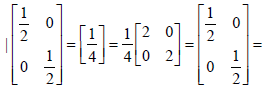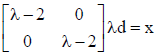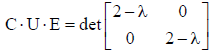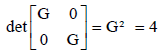Gravity and Electromagnetism | OMICS International
Fluid Mechanics: Open Access
All submissions of the EM system will be redirected to Online Manuscript Submission System. Authors are requested to submit articles directly to Online Manuscript Submission System of respective journal.

# Gravity and Electromagnetism

Paul TE Cusack*

Independent Researcher, BSc E, DULE, 1641 Sandy Point Rd, Saint John, NB, Canada E2K 5E8, Canada

*Corresponding Author:
Cusack P
Independent Researcher, BSc E
DULE, 1641 Sandy Point Rd, Saint John
Tel: (506) 214-3313
E-mail: [email protected]

Received Date: December 10, 2016; Accepted Date: March 18, 2017; Published Date: March 28, 2017

Citation: Cusack PTE (2017) Gravity and Electromagnetism. Fluid Mech Open Acc 4: 155. doi: 10.4172/2476-2296.1000155

Copyright: © 2017 Cusack PTE. This is an open-access article distributed under the terms of the Creative Commons Attribution License, which permits unrestricted use, distribution, and reproduction in any medium, provided the original author and source are credited.

Visit for more related articles at Fluid Mechanics: Open Access

#### Abstract

Here is a paper that uses the theory of electromagnetism to model gravity and the universe. The standard magnetic flux, capacitance, conductivity, dielectrics are considered. The mathematics includes the golden mean parabola, Euler’s Identity, Matrices, Eigenvectors, and Differential equations

#### Keywords

Gravity; Magnetic flux; Capacitance dielectric; Permeability; Mass gap

#### Introduction

Here we provide a solution to the physical universe that shows that it can be modelled as an electromagnetic flux using well know electrical engineering mathematics. The Then gravity is modelled as an eigen vector. The standard Golden Mean parabola and the derivative equals the function is used once again to help solve the problem of why gravity exists. We begin with magnetism and flux.

Magnetic flux density

L=μ0 N²A/l 0.666=1.15 * n² *0.1646/0.8415=√3

B=μ0N i/l=1.15*√ 3 *1.3/0.8415=3.05=c=Mρ

1/L=B/[∂E/∂t]∂M/∂t

∂E/∂t=BL (∂M/∂t]

2t-1=BL (2)

t=5/2=2.5b=T=PERIOD

x³-x-1=2²-2-1=1=E.

Conductivity

R=ρ * l/A

1.618=ρ *0.8415/0.1646

ρ=0.316

σ=1/ρ=3.14=π=E

d=0.8415

d/2=0.42=π-e

Ln e=1

π-Ln e=2.14

2* ∂M/∂t=1 *2=2.

Universal capacitor resistance

Q=1/[ωCRc]

1.3=1/[0.8415 *1.48*Rc]

Rc=1.619 cf 1.618.

Euler's identity

A=E*t=E²=t²=1

i *∫ sin θ -∫cos θ=A=1=-eπ

i* cos π -sin π=1

θ=π=180°

This is half a cycle.

Area Under cosine=0

Area Under sine=π=E.

Capacitance continued

C=q/V

1.5=q/0.86

q=1.3=∂i/∂t where t=1

Now, C=er=e0 A/d

1.5=0.86 (8.854)(A/d)

A/d=√3/8.854

d=0.86 because sin θ=0.86/1

A=0.1956 (0.8415)

A=0.1646

√A=0.4057=t

d0=1/2

0.4057/2=0..2028=Y=E cf Y=0.203 [Dampened Cosine Curve]

t=s-c

t=s-∂s/∂t

Integrate (Time is a Constant with respect to t)

1=s²/2-s

s²-s-1=0

x²-x-1=0

t=s-c

t=s- ∂s/∂t

Integrate: t²/2=s²/2-s.

t=1

t²/2=s²/2-s

2=s²-s

s²-s-2=0

s=2, -1

-∂s/∂t=-1

∂s/∂t=1

∂t/∂t=1

∂s/∂t=s

y=y'

The Derivative Equals the Function

d=vit+1/2 at² (same equation)

d=si+s/2

d=d/2+d/2

si=d/2

The initial distance=1/2

∂E/∂t=2t-1

∂E/∂t=2(1/2)-1=0 (Minimum)

F=v

M=R

V=L ∂i/∂t F=V=v

0.86=0.666 ∂i/d∂t

3/2 *0.666=∂i/∂t

1.3=∂i/∂t

1-∂i/∂t=1-1.3=-0.3=-c/10=-∂s/∂t

current i=speed of light c.

s=t-i 0.1334=t-1.3

t=1.16666

E=1/t=1/1.166=0.86

1-∂i/∂t=∂s/∂t

t-i=s

t-c=s

s=t-c

t=s-c

time=distance-speed of light

distance=time-speed of light/10

distance=0.4+0.3=0.7

1/s=1.4285

s=0.85

V=L ∂i/∂t

But F=G M1M2/R²

∂i/∂t=M1M2/R²

when M1 is very large and M2 is very small,=1/R².

∫ ∂i/∂t=i=∫1/R²=-1/R

i=-1/R

F²/2=-1/R

1/2R² * R=-1

1/2 vM=K.E.=1

Therefore, F=v R=M.

#### Dielectric Constant and Permeability

L'C'=ε μ

(0.666)(1.5)=1 1=ε(15%)

ε=1/1.15=0.86

Capitance=1.48

C*M=1.5*2=3=Mρ

Mass Gap

L=1/1.5=0.6666=G.

So the inductance of our universe is G. The energy of the mass with velocity is converted to a Gravitational field. G=0.666 is the result. Gravity is modelled like a magnetic field. Like magnets, matter is attracted to matter. We call it gravity. The Earth has a magnetic field because it has matter or mass. The magnetic field is proportional to g=9.81.

5.972/9.81=0.609=1/1.642

(The Mass of the Earth is closer to 6.060108)

Better still

1/1.618=0.618 0.618*9.806=6.06=2.02*3=Y*c=Ec

But E=Mc²

So Ec=Mc³=g/M

M²c³=g

(This is why g=9.81m/s)

g=M²Mρ³

g=M² * (∂M/∂t)³

g=E * Mc

a=E *M ∂s/∂t

a=E*M v

a/v=1=E*M

E*M=1=t

M=t².

#### Conservation of Momentum

Mv1=Mv2

v=sin θ

M v1=M sin 1

∂M/∂t *v1=M 0.8415

2 *v1=0.8415M

v1=0.8415/2 *M

=0.4207M

=cuz M

0.8415/cuz=M

M=2

E=Mc²

π=2 c²

c=√(π/2)

c=1.2533=MINIMUM OF THE ENERGY PARABOLA

2t-1=0

t=1/2

P=Mv=2 (0.866)=1.73=√3

K.E.=1/2 Mv²

1=1/2 M (0.86)²

M=2.666

M=-∂M/∂t+G

And

M=F=26.666

Now,

K.E.=1/2 Mv²

Pi=1/2 (2) v²

v²=π

v=√π=1.77

Bernoulli: mgh +1/2mv²+p

0.666b h +1/2 (1.77)²+0

1/h=0.4240=cuz

E/h=cuz Hooke's Law.

limy→0∫xedx=y

limy→0exdy=y

ex=y

y=y'=ex

y=y'=y''=y'''...=ex

lim y==> ∞=ex

eInfinity

x=Ln ∞

y=y'=y''=.....=e^x=∞

The universe is robust [1-4].

#### Similar Matrices

B=S-1 A S

ΩA=√(3/2)

√3/√ 4=[2 0, 0 2]=[√3 v0 0 √3][|A|=3

det |A-1|=1/[det |A|.|D|=1/4

So, eigenvaluesx²-x+ 1/4=4

√3=|A|, 0.7360=√0.858=√Ω A.

#### Mass Gap

E x t x s=E/t

t Ets/E=√3/√4

t²s=√ 3/2

(t²s)²=(√3/√4)²

t4s²=3/E

Et4s²=c

But E=Mc²

M=1/[E t4s²]=1/{√3 * t² s]=1.5 MASS GAP

Aside: E x t x s]/E/t=t²s=(0.4083)(0.866)=0.1444=0.856

C. G. E.=Cusack Gravitational Equation

1/G=M ρ/E ρ+ ∂M/∂t

The mass and energy density is wrt time, not space.

So, ∂²E/∂t²=dE/dt/dM/dt+1/∂M.∂t

(∂E/∂t)²=[∂E/∂t +1]/∂M/∂t

x² ∂M/.∂t -x-1=0

∂M/∂t=2

[∂M/∂t]/2=1

E=Mc²

Mass=T. E=P.E.+K. E..+18=2(3²)

(0.618)³=0.2303

1/(0.618³)=4.236=cuz *10

cuz=π-e.

#### Eigenvalues and Eigenvectors

Eigenvalue of the4-4λ+λ ²=0

x²-4x-4=0

(x-2)(x-2)=0

x=2=λ

A=[2 0, 0 2]

|A|=4=Eρ∂²E/∂t²=E ρ

∂E²/∂t²-E-E ρ=0=Ln t

Now, going back: [2 0, 0 2][x y]=[λ [x y]

[G 0, 0 G] [t E]=G [t E]

Multiply by the diagonal of the unit cube √3/G==1.73/2=0.86

0.86 [2 0 0, 0 2 0, 0 0 2] [1 1 1]]=G [t s E]

√3=2t

t=√3/2=0.86

s=√3/2=0.86

E=√3/2=0.86

∂²E/∂t²- E=Ln t

2-1=Ln t

e2/e1=t

t=et

Ln t=t

Ln t=et

Derivative

1/t=et

So

Ln t=1/t Or the derivative=the function

y=y'

M=mgh+1/2 mv²

1=gh+1/2v²

If v=a

1/2a²+2a-2=0

(a-1)(a-1)=0

a=1

M=P.E.+K. E.

M=mgh+1/2mv²

v=1=a

But a=E=2G

g=1/2

2=2(1/2)(1)+1/2(2)(1²) 2=1+1.

G=2

∂²E/∂t²=2=∂M/∂t

∫∂²E/∂t²=M

∂E/∂t=M

∫∂E/∂t=∫M

E=M²/2

E=1/2 bM²

1/2M²=Mc²

1/2M=c²

M=2c²=18=P.E. +K.E.

1-0.1415=0.8575=0.

y''+y'+y=0

∂²E/∂t²+y'+ E=Ln t

y'=Ln t

y=1/t

E=1/t

d2E/dt2(Ln t)^2/2

dE2/dt2=y''.

So,

(Ln t)²/2+ Ln t+1/t=0

When a=v, this is

1/2 a²+ a+v=0

a²+2a+2v=0

(y'')²+2y''=2y'=0

G²/2+ 2G+2(E')=0

2(2G-1)=-G²/2+2G

4G-2=G²/2+2G

8G-4-4G=G²

4G-4=G²

G²-4G+4=0

(G -2)(G-2)=0

(G -2)^2=0

G=2=∂²E/∂t².

#### Second Order Liners Equations

ay''+by'+cy=0

∂²E/∂t²- (1) E=Ln t

by'=Ln t 0=Ln t

t=1

ar²+br+ c=0

(1)r²=0r+ (-1)=0

r²=1

r=±1

(1)(1)=0(1)=(-1)=0

0=0 true

The Material Universe Exists Where the Gravity Eigenvector.

Eigenvector=1/1.5=0.667 i.e.

1/(3/2) G=E/(Mρ ∂M∂t)

∂²E/∂t²=E/[M ρ/(∂M/∂t)]

where E=x²-x-1

Why does E=1?

2x-1=∫x²-x-1

2x-1=x³/3-x²/2-x-1-C

C=-1 @ x=0

E=(-1)^2-(-1)-1

E=1

E=x^2-x-1=∫ E

E=E'

x²-1=x³/3-1.5x²-x-1=3/2

x³-2.5x²-x-0=1.5

x³-2.5x²-x-1.5=0

x=3=M ρ=c

x=t

This is the solution to our physical universe.

#### Conclusion

We see that the physical universe can be thought of as an electrical flux and gravity as an eigenvector.

#### References

Select your language of interest to view the total content in your interested language

### Article Usage

• Total views: 976
• [From(publication date):
April-2017 - Nov 17, 2019]
• Breakdown by view type
• HTML page views : 886Can't read the image? click here to refresh